# Chapter 3 - Atoms and Molecules Notes | Study Science - Short Notes Class IX - Class 9

## Class 9: Chapter 3 - Atoms and Molecules Notes | Study Science - Short Notes Class IX - Class 9

The document Chapter 3 - Atoms and Molecules Notes | Study Science - Short Notes Class IX - Class 9 is a part of the Class 9 Course Science - Short Notes Class IX.
All you need of Class 9 at this link: Class 9

Elements

The purest form of matter that are made up of a single type of atoms. E.g. Carbon (C), Oxygen (O).

Compounds

Compounds are made up of two or more type of elements combined chemically in fixed ratio by their masses. E.g. Carbon dioxide (CO2).

Atoms

The smallest constituent particles of matter that cannot be broken down further. E.g. Atom of oxygen is O, nitrogen N

Molecules

The form of matter made up of two or more atoms that can exist independently. E.g. Oxygen and nitrogen can exist as single atom. They combine with another atom of their own kind to form a molecule of Oxygen i.e. O2 and nitrogen i.e. N2.

Laws of Chemical Combination

(i) Law of Conservation of mass : Mass can neither be created nor be destroyed. It can only be converted from one form to another.
(ii) Law of Constant Proportion : Two or more elements combine in fixed proportions (ratio) by their mass to form a compound.
Example

•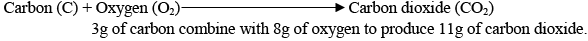• Similarly, 9g of water decomposes to produce 8g of oxygen and 1g of hydrogen.

Dalton’s Atomic Theory

• Matter is made of tiny particles called atoms.
• Atoms can neither be created or be destroyed.
• All the atoms of an element have same mass and chemical properties.
• Atoms of different element have different atomic mass and chemical properties.
• Atoms combine in the ratio of whole numbers to form compounds.
• The relative number and kinds of atoms are constant in a given compound.

Atoms

• The smallest constituent particles of matter that cannot be broken down further. E.g. Atom of oxygen is O, nitrogen N.
• However, an atom is made up of protons, neutrons and electrons.
• Atoms are very small.
• Radius of hydrogen atom = 10-10m
• Radius of a water molecule = 10-9m

➢ Symbols of elements

• Various elements have been assigned a symbol, which is specific to that element and is used refer to it in various reactions.
• Dalton was the first scientist to use symbols

➢ IUPAC or International Union For Pure and Applied Chemistry approves the symbols for elements.
➢ Atomic Number : Number of protons or electrons in an uncharged atom is known as its atomic number.
➢ Atomic mass : Number of protons and neutrons in an atom is called its atomic mass. Its unit is atomic mass unit (amu or u).
One atomic mass unit is defined as the one twelfth (1/12th) the mass of one carbon-12 atom.
➢ For e.g. The atomic mass of oxygen atom is 16. It means that it is 16 times heavier than one twelfth (1/12th) the mass of one carbon-12 atom.

➢ Some common elements and their atomic number and masses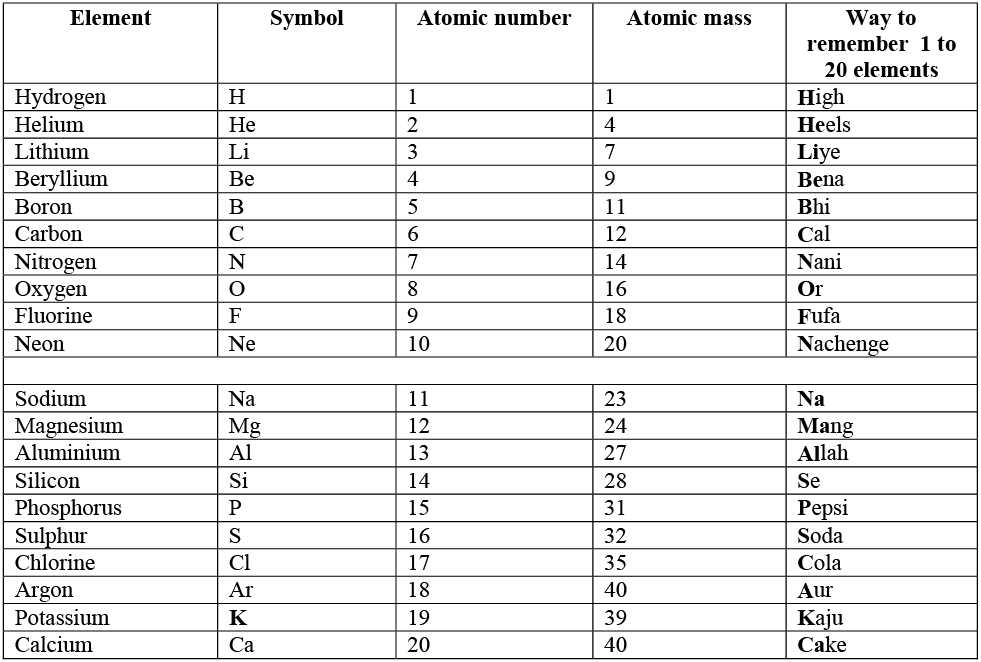Molecule

The form of matter made up of two or more atoms that can exist independently. E.g. Oxygen and nitrogen can exist as single atom. They combine with another atom of their own kind to form a molecule of Oxygen i.e. O2 and nitrogen i.e. N2.

➢ Molecules of elements
(i) Molecules of element – made of one or more atoms of the same element combined chemically to form a stable substance.
Some elements usually non-metals are very reactive at normal conditions and hence react with more atom/s of their own kind to form a molecule.

• Atomicity : Number of atoms in a molecule.
• Monoatomic : A molecule that contain only one atom
• Diatomic : A molecule that contain two atoms
• Triatomic : A molecule that contain 3 atoms
• Tetra-atomic :  Molecule that contain four atoms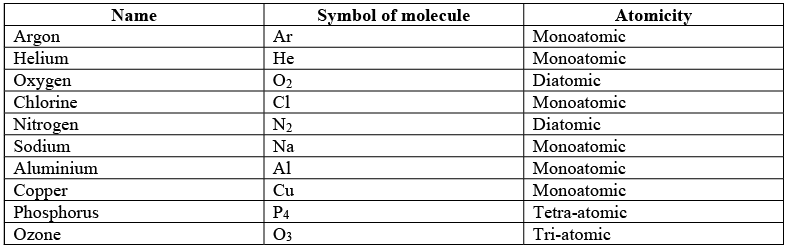(ii) Molecules of Compounds : Compounds are made up of two or more type of elements combined chemically in fixed ratio by their masses. E.g. Carbon dioxide (CO2).

• Ratio mass of Carbon and Oxygen in CO2 = 12 / 16 * 2 = 3 : 8
(16 * 2 because atomic mass of oxygen is 16 and there are two atoms of oxygen in CO2)
• Water – H2O; Ratio of hydrogen and oxygen in H2O = 1 * 2 / 16 = 1 : 8

Ion

Charged species are called ions

• Atoms are made up of electrons, protons and neutrons.
• Electrons are negatively charged; protons are positively charged while neutrons do not carry any charge.
• Protons and neutrons are present in the center, in a nucleus and electron revolve around the nucleus.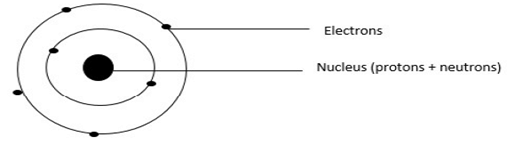An atom of carbon

The number of electron and protons is same in an uncharged atom. For example, Sodium’s atomic number is 11 and an uncharged atom of sodium contain 11 electrons as well as 11 protons.
However, an atom can gain electrons to become negatively charged and a loose electron to become positively charged.
Explanation : There are 11 electrons and 11 protons in a neutral sodium atom. If sodium loses one electron, the number of proton remain 11 and the number of electrons reduces to 10. Since, there is one more positive charge than negative sodium becomes positively charged.
That can be written as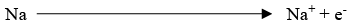Sodium produces sodium ion on losing one electron.

➢ Types of ions
(i) Cations : A positively charged ion is called cation. Cations are formed when an atom/molecule loses electron. Metals usually form cations.
(ii) Anions : A negatively charged ion is called anion. Anions are formed when an atom/molecule gains electrons.

Chemical Formula

The symbolic representation of the composition of elements in a compound is called its chemical formula.
➢ Valency : The combining capacity of an element of molecule is called its valency.
Valency of some Ions: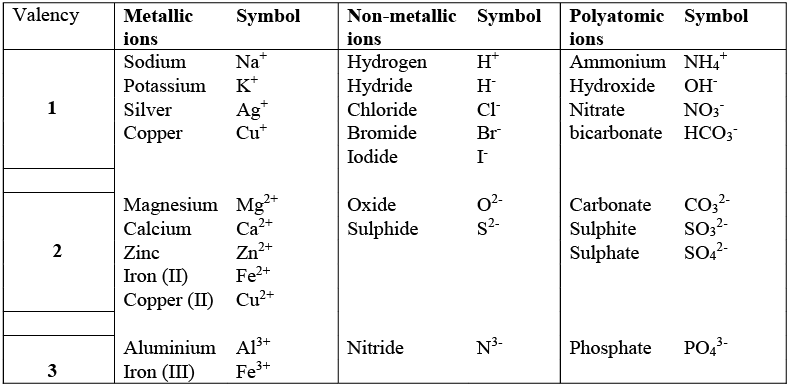➢ Rules to write a chemical formula

• Valencies of the ion should be balanced (the compound should not have any charge as a whole).
• If a compound consists of a metal and a non-metal, name of symbol of the metal should be written first followed by the name or symbol of the non-metal.
• If the compound is formed from polyatomic ion, the ions should be enclosed in a bracket before writing the ratio.

➢ Examples
(i) Formula of sodium chloride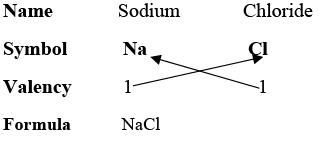(ii) Formula for Calcium chloride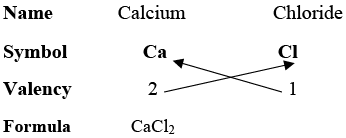(iii) Formula for Calcium oxide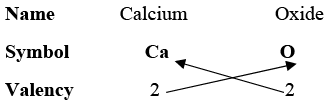Formula             Ca2O2 but the formula is always written in the simplest ratio and 2 / 2 = 1 : 1 so Formula for calcium chloride is CaO.
(iv) Formula for Ammonium carbonate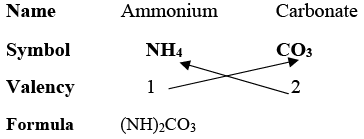Molecular Mass and Mole concept

➢ Molecular mass : Sum of atomic masses of all the atoms present in a molecule.
For e.g. Atomic mass of Na2O = 2 x atomic mass of Na + atomic mass of oxygen
= 2 x 23 + 16 = 62u.
➢ Formula unit mass : Sum of atomic masses of all the atoms present in a formula unit or empirical formula of that compound.
Empirical formula of a compound represents the elements of a compound in their simplest ratio regardless of their chemical composition.
E.g.  Disulphur dioxide (S2O2) contains two atoms of both sulphur and oxygen.
The empirical formula for disulphur dioxide (S2O2) is SO as the simplest ratio of 2 : 2 is 1 : 1.
Molecular mass of disulphur dioxide = 2 * atomic mass of sulphur + 2 * atomic mass of oxygen
= 2 * 32 + 2 * 16
= 96u
Formula unit mass or empirical mass of disulphur dioxide (S2O2)
Empirical formula of disulphur dioxide = SO
Empirical or formula unit mass = 32 + 16 = 48u
However, the formula unit mass and the molecular mass for compound which are already in the simplest ratio remains the same.
➢ Mole concept : Mole is a unit. One mole of an atom or molecule is its atomic or molecular mass of that molecule in grams.
The number of molecules in one mole of a substance is always fixed i.e. 6.022 * 1023 or the Avogadro number.
The value 6.022 * 1023 is known as Avogadro’s number.
➢ Example
(i) Atomic mass of Sodium is 23u
Mass of one mole of sodium will be 23g and
One mole of sodium will also contain 6.022 * 1023 molecules of sodium.
(ii) Calcium chloride (CaCl2
Molecular mass of calcium chloride = 40 + 2 * 35.5 = 111u
Molar mass of CaCl2 = 111g
No. of molecules in 1mole of CaCl2 = 6.022 * 1023 molecules.
Mathematical calculations to find the mole and number of atoms can be done by unitary method or the following formulas:
(i) Number of moles = Given mass / Molar mass
(ii) Number of moles = Given number of particles / Avogadro number

The document Chapter 3 - Atoms and Molecules Notes | Study Science - Short Notes Class IX - Class 9 is a part of the Class 9 Course Science - Short Notes Class IX.
All you need of Class 9 at this link: Class 9Use Code STAYHOME200 and get INR 200 additional OFF

## Science - Short Notes Class IX

13 docs

Track your progress, build streaks, highlight & save important lessons and more!

,

,

,

,

,

,

,

,

,

,

,

,

,

,

,

,

,

,

,

,

,

;